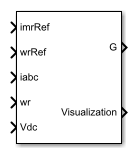# Induction Machine Field-Oriented Control

Per-unit discrete-time induction machine FOC

•Libraries:
Simscape / Electrical / Control / Induction Machine Control

## Description

The Induction Machine Field-Oriented Controller block implements an induction machine field-oriented control (FOC) structure using the per-unit system. To decouple the torque and flux, FOC uses the rotor d-q reference frame. The figure shows the control structure.In the diagram:

• ωr is the measured angular velocity.

• ωref is the reference angular velocity.

• id and iq are the d- and q-axis stator currents.

• ia, ib, and ic are the a-, b- and c-phase stator winding currents.

• imr_ref is the reference magnetizing current.

• imr is the magnetizing current.

• vd and vq are the d- and q-axis stator voltages.

• va, vb, and vc are the a-, b- and c-phase stator winding voltages.

• θe is the rotor electrical angle.

• GAH, GAL, GBH, GBL, GCH, and GCL are the a-, b- and c-phase high (H) and low(L) gate pulses.

### Assumptions and Limitations

• The machine parameters are known.

• The implementation uses the per-unit system.

• The control structure implementation uses a single sample rate.

## Ports

### Input

expand all

Magnetizing reference current in the per-unit system.

Data Types: `single` | `double`

Rotor reference velocity in per-unit system.

Data Types: `single` | `double`

Measured phase currents in the per-unit system.

Data Types: `single` | `double`

Measured angular velocity in per-unit system.

Data Types: `single` | `double`

Data Types: `single` | `double`

### Output

expand all

Inverter gate pulses. The block does not consider any dead time.

Data Types: `single` | `double`

Bus containing signals for visualization.

Data Types: `single` | `double` | `bus`

## Parameters

expand all

### General

Nominal voltage.

Nominal electrical frequency.

Rotor, stator-side resistance in the per-unit system.

Rotor stator-side leakage inductance, in the pu-unit system.

Magnetizing inductance in the per-unit system.

Time constant for filtering the d and q currents.

Voltage threshold to activate the power inverter.

Fundamental sample time must be less than the control sample time.

Control sample time must be greater than the fundamental sample time.

### Outer Loop

Proportional gain for the magnetizing current controller.

Integral gain for the magnetizing current controller.

Integral anti-windup gain for the magnetizing current controller.

Proportional gain for the speed controller.

Integral gain for the speed controller.

Integral anti-windup gain for the speed controller.

Maximum current for the d-axis.

Maximum current for the q-axis.

Inner Loop

Align the a-phase vector of the abc reference frame to the d- or q-axis of the rotating reference frame.

Proportional gain of the PI controller used for direct-axis current control.

Integrator gain of the PI controller used for direct-axis current control.

Anti-windup gain of the PI controller used for direct-axis current control.

Proportional gain of the PI controller used for quadrature-axis current control.

Integrator gain of the PI controller used for quadrature-axis current control.

Anti-windup gain of the PI controller used for quadrature-axis current control.

Prioritize or maintain ratio between d- and q-axes when block limits voltage.

PWM

Specify the waveform technique.

The sampling mode determines whether the block samples the modulation waveform when the waves intersect or when the carrier wave is at one or both of its boundary conditions.

Specify the rate at which you want the switches in the power converter to switch.

## Version History

Introduced in R2017b